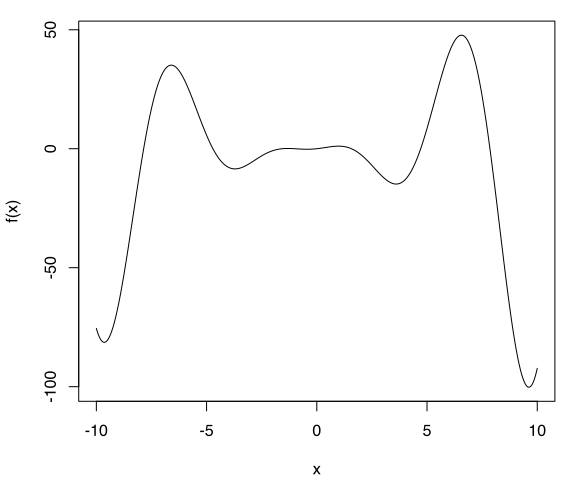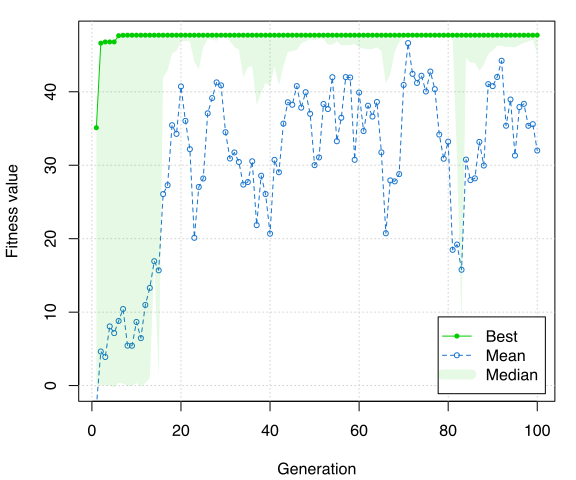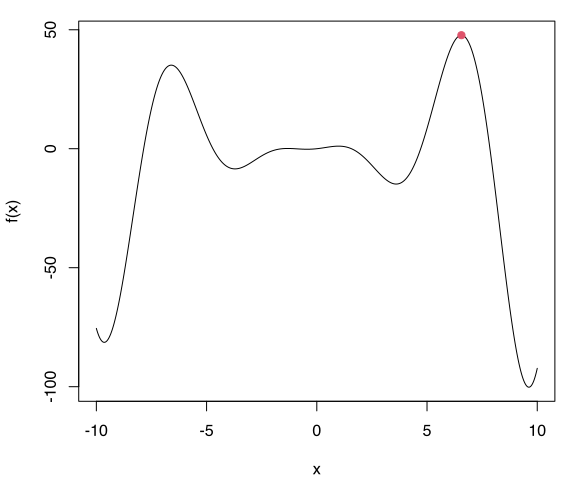# Introduction

Genetic algorithms (GAs) are stochastic search algorithms inspired by the basic principles of biological evolution and natural selection. GAs simulate the evolution of living organisms, where the fittest individuals dominate over the weaker ones, by mimicking the biological mechanisms of evolution, such as selection, crossover and mutation.

The R package GA provides a collection of general purpose functions for optimization using genetic algorithms. The package includes a flexible set of tools for implementing genetic algorithms search in both the continuous and discrete case, whether constrained or not. Users can easily define their own objective function depending on the problem at hand. Several genetic operators are available and can be combined to explore the best settings for the current task. Furthermore, users can define new genetic operators and easily evaluate their performances. Local search using general-purpose optimisation algorithms can be applied stochastically to exploit interesting regions. GAs can be run sequentially or in parallel, using an explicit master-slave parallelisation or a coarse-grain islands approach.

This document gives a quick tour of GA (version 3.2.3) functionalities. It was written in R Markdown, using the knitr package for production. Further details are provided in the papers Scrucca (2013) and Scrucca (2017). See also help(package="GA") for a list of available functions and methods.

library(GA)
##   ____    _
##  / ___|  / \     Genetic
## | |  _  / _ \    Algorithms
## | |_| |/ ___ \
##  \____/_/   \_\  version 3.2.3
## Type 'citation("GA")' for citing this R package in publications.

# Function optimisation in one dimension

Consider the function $$f(x) = (x^2+x)\cos(x)$$ defined over the range $$-10 \le x \le 10$$:

f <- function(x)  (x^2+x)*cos(x)
lbound <- -10; ubound <- 10
curve(f, from = lbound, to = ubound, n = 1000)GA <- ga(type = "real-valued", fitness = f, lower = c(th = lbound), upper = ubound)
summary(GA)
## ── Genetic Algorithm ───────────────────
##
## GA settings:
## Type                  =  real-valued
## Population size       =  50
## Number of generations =  100
## Elitism               =  2
## Crossover probability =  0.8
## Mutation probability  =  0.1
## Search domain =
##        th
## lower -10
## upper  10
##
## GA results:
## Iterations             = 100
## Fitness function value = 47.70561
## Solution =
##           th
## [1,] 6.55988
plot(GA)curve(f, from = lbound, to = ubound, n = 1000)
points(GA@solution, GA@fitnessValue, col = 2, pch = 19)# Function optimisation in two dimensions

Consider the Rastrigin function, a non-convex function often used as a test problem for optimization algorithms because it is a difficult problem due to its large number of local minima. In two dimensions it is defined as $f(x_1, x_2) = 20 + x_1^2 + x_2^2 - 10(\cos(2\pi x_1) + \cos(2\pi x_2)),$ with $$x_i \in [-5.12, 5.12]$$ for $$i=1,2$$. It has a global minimum at $$(0,0)$$ where $$f(0,0) = 0$$.

Rastrigin <- function(x1, x2)
{
20 + x1^2 + x2^2 - 10*(cos(2*pi*x1) + cos(2*pi*x2))
}

x1 <- x2 <- seq(-5.12, 5.12, by = 0.1)
f <- outer(x1, x2, Rastrigin)
persp3D(x1, x2, f, theta = 50, phi = 20, col.palette = bl2gr.colors)